• java语言编写的十进制正数和负数转换二进制算法。
• Java十进制到二进制转换的3种不同方法。 1. Integer.toString（）方法 我们可以使用Integer类的toString（）方法将十进制数转换为二进制数。此方法有两个参数，第一个参数是十进制数，第二个参数是我们要转换的...
Java十进制到二进制转换的3种不同方法。

1. Integer.toString（）方法

我们可以使用Integer类的toString（）方法将十进制数转换为二进制数。此方法有两个参数，第一个参数是十进制数，第二个参数是我们要转换的基数。对于二进制，基数为2。

1

2

3

4

5

6

7

8

9

10

11

12

13

import java.util.Scanner;

public static void main(String args[]){

int number;

Scanner sc = new Scanner(System.in);

System.out.println("Enter a decimal number: ");

number = sc.nextInt();

System.out.println("Binary number is " + Integer.toString(number, 2));

}

}

输出量

输入十进制数字：8二进制数字为1000

2. Integer.toBinaryString（）

这是Integer类的另一种方法，可以直接将十进制转换为二进制。请参阅下面的程序以了解如何使用它。

1

2

3

4

5

6

7

8

9

10

11

12

13

import java.util.Scanner;

public static void main(String args[]){

int number;

Scanner sc = new Scanner(System.in);

System.out.println("Enter a decimal number: ");

number = sc.nextInt();

System.out.println("Binary number is " + Integer.toBinaryString(number));

}

}

输出量

输入十进制数字：10二进制数字为1010

3.自己的逻辑

我们可以在Java中编写自己的逻辑，将十进制转换为二进制。可以通过以下步骤完成。

使用％运算符将数字除以2，并将剩余的数存储在某个位置。
使用/运算符将数字除以2。
重复上述两个步骤，直到数字变为0。
现在，以相反的顺序打印其余部分。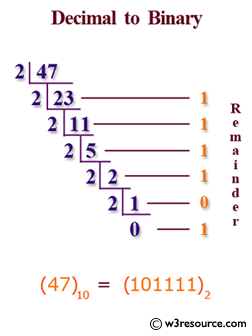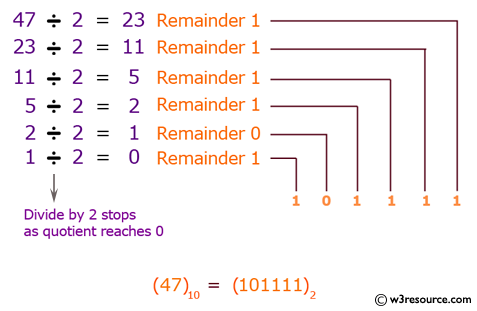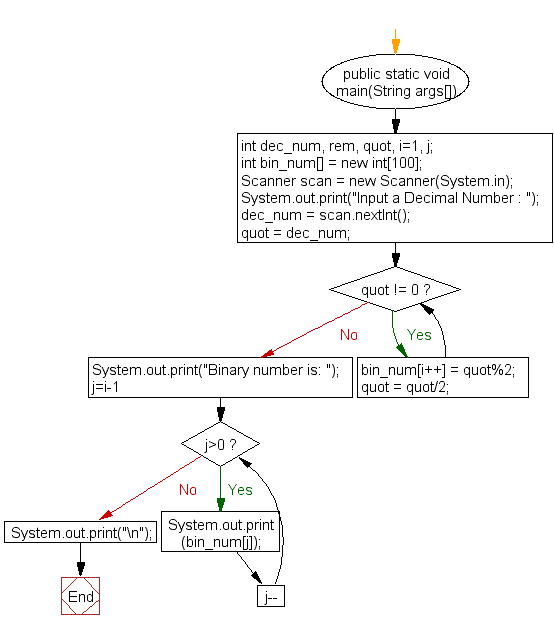下面的程序实现了这个简单的算法。

1

2

3

4

5

6

7

8

9

10

11

12

13

14

15

16

17

18

19

20

21

22

23

24

25

26

import java.util.ArrayList;

import java.util.Collections;

import java.util.Scanner;

public static void main(String args[]){

int number;

Scanner sc = new Scanner(System.in);

ArrayList<String> binary = new ArrayList<String>();

System.out.println("Enter a decimal number: ");

number = sc.nextInt();

while(number != 0){

number /= 2;

}

Collections.reverse(binary);

System.out.print("Binary number is ");

for(String s : binary){

System.out.print(s);

}

}

}

输出量

输入十进制数字：2二进制数字为10


展开全文• java二进制转化为十进制Here you will get program to convert binary to decimal in Java. 在这里，您将获得在Java中将二进制转换十进制的程序。 There are mainly two ways to convert a binary number to ...java二进制转化为十进制Here you will get program to convert binary to decimal in Java.
在这里，您将获得在Java中将二进制转换为十进制的程序。
There are mainly two ways to convert a binary number to decimal number in Java.
在Java中，主要有两种将二进制数转换为十进制数的方法。
1. By using parseInt() method of Integer class.
2. By using user defined logic.
1.通过使用Integer类的parseInt()方法。 2.通过使用用户定义的逻辑。
用Java将二进制转换为十进制的程序 (Program to Convert Binary to Decimal in Java)
By using Integer.parseInt()
通过使用Integer.parseInt()
Integer.parseInt() method takes two arguments. First argument is a string and second argument is the base or radix in which we have to convert the number. The output is the integer represented by the string argument in the specified radix. Below is the program for it.
Integer.parseInt()方法采用两个参数。 第一个参数是一个字符串，第二个参数是我们必须在其中转换数字的基数或基数。 输出是由指定基数中的字符串参数表示的整数。 下面是它的程序。
import java.util.Scanner;

class BinaryToDecimal
{
public static void main(String args[])
{
Scanner s=new Scanner(System.in);

System.out.println("Enter a binary number:");

String n=s.nextLine();

System.out.println(Integer.parseInt(n,2));
}
}
Without using Integer.parseInt()
不使用Integer.parseInt()
In this method we have to define our own logic for converting binary number to decimal. The approach that we will use here is mentioned in below example.
在这种方法中，我们必须定义自己的逻辑，以将二进制数转换为十进制。 下面的示例中提到了我们将在此处使用的方法。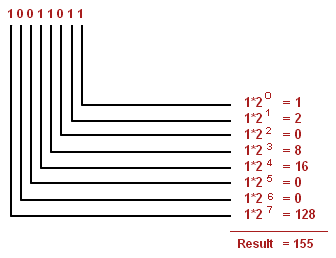Image Source
图片来源
The program that implements above approach is mentioned below.
下面介绍实现上述方法的程序。
import java.util.Scanner;

class BinaryToDecimal
{
public static void main(String args[])
{
Scanner s=new Scanner(System.in);

System.out.println("Enter a binary number:");
int n=s.nextInt();

int decimal=0,p=0;

while(n!=0)
{
decimal+=((n%10)*Math.pow(2,p));
n=n/10;
p++;
}

System.out.println(decimal);
}
}
Output
输出量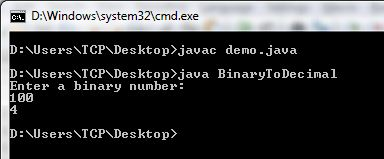If you found anything missing or incorrect in above programs then please mention it by commenting below.
如果您在上述程序中发现任何丢失或不正确的内容，请在下面的评论中提及。
翻译自: https://www.thecrazyprogrammer.com/2015/11/program-to-convert-binary-to-decimal-in-java.htmljava二进制转化为十进制
展开全文python 编程语言 spring
•代码
• 1.十进制整数转二进制 思路： 整数除以2，每次将余数保存起来，商循环除以2，直到商为0为止，然后将所有余数逆序打印出来。 例子： 23/2 商: 11 余数: 1 11/2 商: 5 余数: 1 5/2 商: 2 余数: 1 2/2 商: 1 余数:...
1.十进制整数转二进制

思路： 整数除以2，每次将余数保存起来，商循环除以2，直到商为0为止，然后将所有余数逆序打印出来。

例子：

23/2  商: 11  余数: 1
11/2  商: 5   余数: 1
5/2    商: 2   余数: 1
2/2    商: 1   余数: 0
1/2    商: 0   余数: 1

 //十进制整数转二进制，每次取商除以2直至商为0，余数追加，最后倒序一下
public static StringBuilder intToBinary(int n){
StringBuilder builder = new StringBuilder();
//商
int quotient = n;
//余数
int remainder =0;
while(quotient!=0){
quotient = n/2;
remainder = n%2;
builder.append(remainder);
n = n/2;
}
return builder.reverse();
}

2.十进制小数转二进制

思路： 小数乘以2，每次乘完取整（1或者0）放到数组中，然后将剩余小数部分继续乘以2，直到小数部分为0或者达到所要求的精度为止，再讲所有余数按顺序打印出来。

例子：

0.813*2   整数部分: 1  小数部分: 0.626
0.626*2   整数部分: 1  小数部分: 0.252
0.252*2   整数部分: 0  小数部分: 0.504
0.504*2   整数部分: 1  小数部分: 0.008
0.008*2   整数部分: 0  小数部分: 0.016

11010是精度为5的小数点后五位，输出为0.11010

注：小数的十进制转化为二进制的误差不大于2^-4是指小数点后4位

代码实现：

 //十进制小数转成二进制,d是十进制的小数，decimal是精度
public static StringBuilder doubleToBinary(Double d,int decimal) throws Exception{
if(decimal<=0 || decimal>32){
throw new Exception("精度不合法");
}
StringBuilder str = new StringBuilder();
double rem = 0;
while(decimal>0){
rem = d*2;
if(rem>=1){
str.append(1);
d = rem -1;
}else{
str.append(0);
d = rem;
}
decimal--;
}
return str;
}

3.十进制的整数加小数转二进制

思路： 整数和小数分别转换，然后将两个数组以小数点为分割分别打印出来。
例子：

代码实现：

public static StringBuilder allToBinary(double d) throws Exception{
int in = (int)d;//整数部分
double res = d - in;//小数部分
StringBuilder str = new StringBuilder();
str.append(intToBinary(in));
str.append(".");
str.append(doubleToBinary(res,4));
return str;
}

调用和运行结果：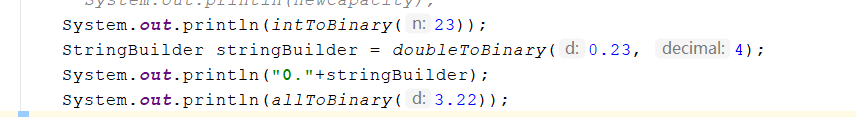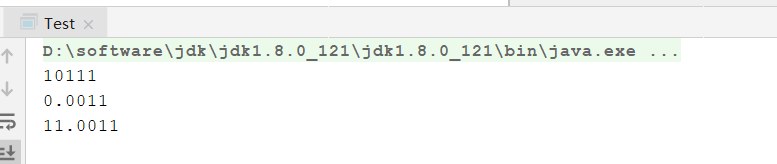展开全文• 1.十进制转成二进制 String s = Integer.toBinaryString(n) //将十进制数转成字符串，例如n=5 ，s = "101" 2.将字符串转成整形 int a = Integer.valueof("1002"); //当然s只能是数字类的字符串 或者 int a = ...
1.十进制转成二进制

String s = Integer.toBinaryString(n)  //将十进制数转成字符串，例如n=5 ，s = "101"

2.将字符串转成整形

int a = Integer.valueof("1002");  //当然s只能是数字类的字符串

或者

int a = Integer.parseInt("1002");

3.将整形转成字符串
String s = String.valueof(1025);  直接转成了

4.将整形转成十六进制的数

String s = Integer.toHexString(18);   //输出结果12


展开全文• * 十进制--->二进制 */ public static void toBin1(int num){ trans(num, 1, 1); } /* * 十进制--->八进制 */ public static void toBa(int num){ trans(num, 7, 3); } /* * 十进制--->十六进制 */ ...
• 十进制数转二进制数，跟据不同的开发语言其转换方式有很多，在Java中如果相把一个十进制的整数来转换二进制那是举手之劳，非常简单，只要用Integer.toBinaryString(int)方法就可以得到结果。但如果转换的不是一个...算法
• java十进制进制转换之移位，看了一些然后自己理解了一下现在就详细的讲解一下。 首先你得直到计算机内的存储都是采用0和1,是二进制序列来表示图像数字文字等等。 那么一个整数中正数是原码表示，负数和零用补码...binary shift
• 方法一：使用java自代的Integer方法 代码如下： ... //十进制转换二进制 System.out.println(Integer.toBinaryString(i)); //十进制转换为八进制 System.out.println(Integer.toOctalString(i)); ...后端
• 最近由于工作上的需要，遇到进制转换的问题。涉及到的进制主要是 十进制，十六进制，二进制中间的转换。还包括了一个字节数组 与十六进制字符串的互相转换。估计遇到蓝牙通信的朋友，应该用的上。
• A、十进制转换其他 　十进制转成二进制 Integer.toBinaryString(int i) 　十进制转成八进制 Integer.toOctalString(int i)八进制 转换
• //输入一个整数，输出该数二进制表示中1的个数。其中负数用补码表示。 public static void main(String[] args) { Scanner s = new Scanner(System.in); System.out.print(“请输入一个数：”); int n = s....
• public class JinZhiZhuanHua { ... //使用查表法将十进制转换二进制、八进制、十六进制 int num=60; toBin(num); tooct(0); toHex(60); } //转换为二进制 public static void toBin(in
• int i= 28; //十进制二进制 String binaryString = Integer.toBinaryString(i); System.out.println("十进制"+i+"转二进制: "+ binaryString); //二进制十进制 int anInt = Integer...
• /**  * 将十进制转换二进制数  *  * @author wangli  * @param args  */  public static void main(String[] args) {  Scanner input=new Scanner(System.instring input
• 本文记录小编遇到的一种10进制转换为16进制的一种算法。 因为小编能力有限，所以只能做出来0到255之间的十进制数转换为十六进制数。 class HexaDecimal { public static void main(String[] args) { int i1=60;/...
• Java十进制到十六进制的转换：Integer.toHexString()Integer.toHexString()方法将十进制转换为十六进制。 toHexString()方法的签名如下：public static String toHexString(int decimal)让我们看...
• 十进制转换二进制 将二进制转换为十进制 1. 将十进制转换二进制： 思路：对十进制的数进行除2取余法： /** * 讲10 进制转化为二进制 * @param de ：待转换的十进制 * @return ：转换后的二进制（string）...
• package day11;... * 输入一个数字n 输出要求的无符号的二进制串 * */ public class BinaryNumber { public static void main(String[] args) { Scanner scan = new Scanner(System.in); int[] binary...算法
• 1.取余法 public static void TenToBinary(int num){ int r = num%2;//余数 if(num >=2){ TenToBinary(num/2); } System.out.print(r); } 2.java内置函数 Integer.toBinaryString();
• 十进制转成十六进制：Integer.toHexString(int i)十进制转成八进制Integer.toOctalString(int i)十进制转成二进制Integer.toBinaryString(int i)十六进制转成十进制Integer.valueOf("FFFF",16).toString()八进制转成...
• import java.math.BigInteger; /** * @author 进制转换 * */ public class cons { ... @SuppressWarnings("unused") ... // TODO 十六进制转换十进制 long result = 0; for(int i=(serial.length-1);i>=0;i-字符串
•代码
•代码...

# java十进制转换二进制java 订阅